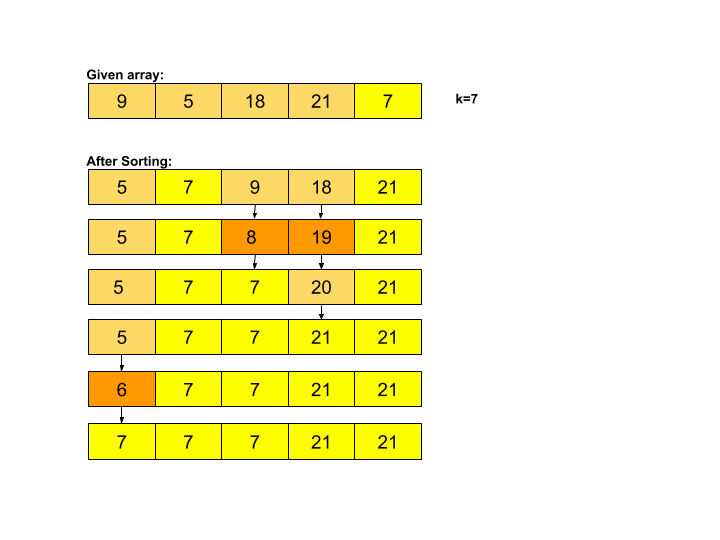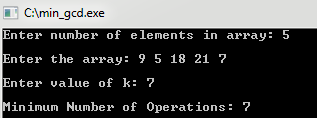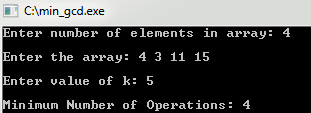×

Search anything:

# Minimum number of operations to make GCD of an array K

#### Algorithms List of Mathematical AlgorithmsGet this book -> Problems on Array: For Interviews and Competitive Programming

We are given an array and we need to find the minimum number of operations required to make GCD of the array equal to k. Where an operation could be either increment or decrement an array element by 1.

Sample Input: ar = {5, 9, 16}, k = 5
Sample Output: 2
Explanation: Note that if we increment 9 by 1 and decrement 16 by 1(no. of operation = 2), the new array will be {5, 10, 15} and hence the GCD will be 5.

# What is a GCD?

So, GCD stands for Greatest Common Divisor which is the greatest integer that divides given two or more integers(not all zero) and leaves 0 as remainder. For example, the GCD of 15(5 * 3 * 1) and 10(5 * 2 * 1) is 5.

It looks like in order to change the GCD to k, we will need to shift every array element towards the closest multiple of k. Now, how are we going to do this?
See, by shifting we basically mean applying a bunch of increment or decrement operations altogether such that we can move in larger steps, say x (x>=1). Next up is moving to the "nearest" multiple. How could we decide this?

Yes, we will be comparing the difference between the possible multiple and the element itself.(Note that for any element ele, there would be two choices for the nearest multiple of k. Those two choices being the greatest multiple of k less than ele and lowest multiple of k greater than ele ).As per the problem, we are now left to figure out these two differences with ele in order to select x(no. of operation) such that it is minimum.

We can easily claim that the two possible solution for x are none other than ele % k and k - ele % k (where ele % k is remainder value we will get on dividing ele by k and k - ele % k indeed is possible difference from the next multiple). So aren't we done now? we could just chose the minimum of two values?

No,Think of a case when arr = {11, 30, 19} and k = 5 (i.e., minimum element in arr > k). Here, after doing the above steps, we will get the array as {10, 30, 20} but are we successful? No, GCD has changed to 10 instead of 5.

Seems like we are stuck. What could we do in order to keep the GCD as k even if the array elements shift towards a multiple of k which is not 1?

You could make an important note here that the maximum value, a GCD can hold is none other than the value of minimum element in the array.

So, taking this as the hint, we could change the minimum element to k, that will make sure that we don't shift GCD beyond k.(because the highest common factor between the array elements will be k only)

# Pseudocode

``````1. Sort the array
2. For i = 1 to n - 1
3.   No_of_operations += min(arr[i] % k, k - arr[i] % k)
4. If arr > k,
5.      No_of_operations += arr - k
else
6.      No_of_operations += k - arr
``````

Consider this example:where arr = {9, 5, 18, 21, 7} and k = 7

Note that going by the steps, we first sorted the array and hence changed arr to {5, 7, 9, 18, 21}. Now, it is the turn we check and change arr[i] to suitable multiple of 7 where i ∈ {1,..,n}.

For the second element(i = 1) in array, since min(7 % 7, 7 - (7 % 7 )) = min(0, 7) = 0. Therefore, No_of_operations = 0

For the third element(i = 2), we noticed min(9 % 7, 7 - (9 % 7)) = min(2, 5) = 2. Therefore, No_of_operations = 2

For the fourth element, min(18 % 7, 7 - (18 % 7)) = min(4, 3) = 3.Therefore, No_of_operations = 5(3 + 2)

For the fifth element, min(21 % 7, 7 - (21 % 7)) = min(0, 7) = 0.Therefore, No_of_operations remain 5

Now as 5 < 7(arr < k).Therefore, No_of_operations = 7(5 + 2)
And we get, minimum number of operations as 7.

Learn other interesting Mathematical Algorithms for other algorithmic problems 😊

# Implementation

``````#include <bits/stdc++.h>
using namespace std;
int main()
{
int n; // no. of elements in array
int k; // new GCD
cout << "Enter number of elements in array: ";
cin >> n;
cout << "\nEnter the array: ";
int arr[n]; // array
for(int i = 0 ; i < n ; i++)
cin >> arr[i];
cout << "\nEnter value of k: ";
cin >> k;
if(k == 0)
{
cout << "\nInvalid value for gcd";
return 0;
}
sort(arr, arr + n);
int min_operation = 0;
for(int i = 1 ; i < n ; i++)
min_operation += min(arr[i] % k, k - (arr[i] % k));
//shift towards closest multiple
if(arr > k)
min_operation += arr - k;
else
min_operation += k - arr;
cout << "\nMinimum Number of Operations: " << min_operation;
return 0;
}
``````

Important note:

Since we are calculating remainder values, we need to take care of "Divide by Zero Error" that might arise when k = 0. As in the code, we can simple check for k and claim it "Invalid" if it is zero. It doesn't make sense for GCD to be equal to 0 right.

### Sample I/O### Complexity

• Time complexity: `Θ(N logN)`
• Space complexity: `Θ(1)`

With this article at OpenGenus, you must have the complete idea of the algorithm to find the minimum number of increment and decrement operations to make GCD of an array 'k'. Enjoy.

#### Shweta Bhardwaj

I panic and hence I exist | Intern at OpenGenus | Student at Indraprastha College for Women, University of Delhi